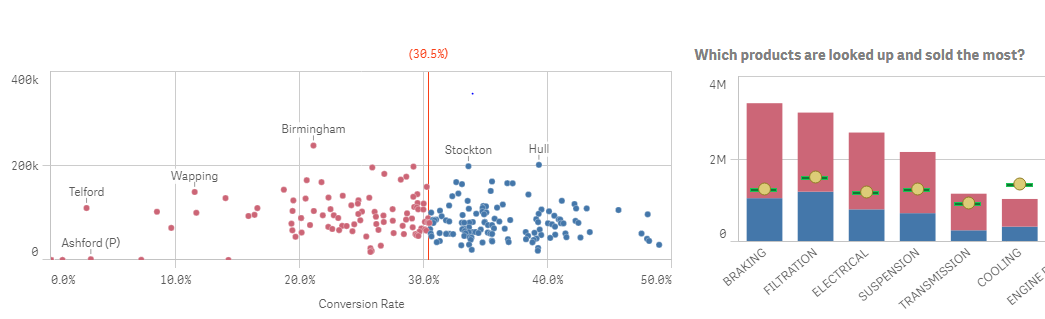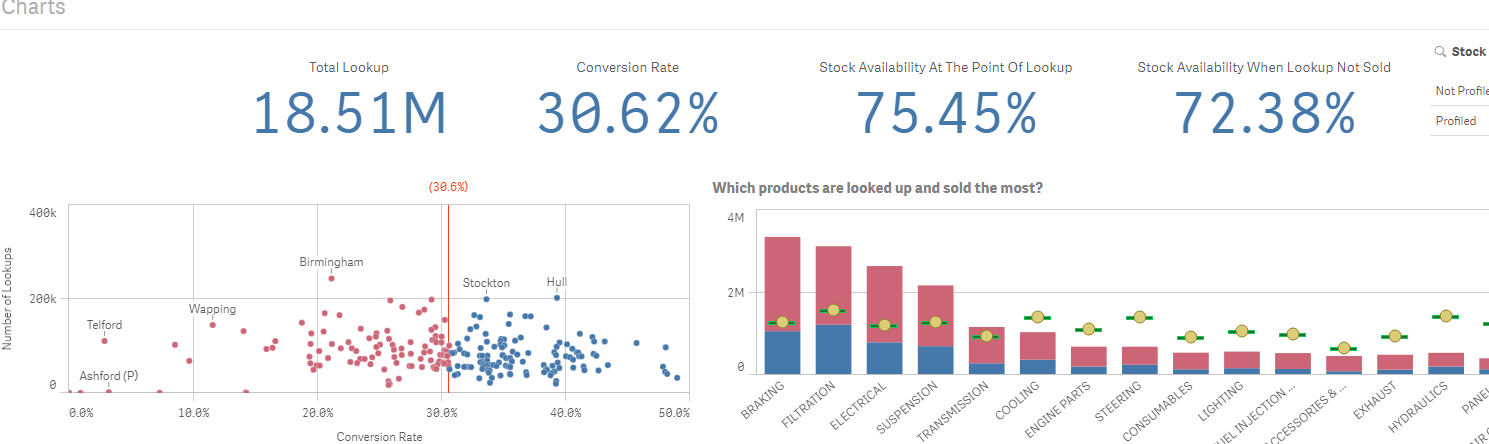Announcements
Sept. 27, 10AM ET Do more with Qlik, Insight Advisor – our intelligent AI-assistant in Qlik Sense: Register
cancel
Showing results for
Did you mean:Contributor

## How to use colors based on set analysis inside if condition?

I am using colors in my scatterplot depending on the compariosion result between branch Conversion Rate vs Company Conversion Rate.

But it is not working. It works when I hard code it.

My Branch conversion rate is a field from table and company conversion rate is derived using set analysis in color section of appearance.

This doesn't work:

if(CR_Branch > Count({<[Converted]={"Converted"}>}[Converted])/Count(HSSRecordID), rgb(68,119,170),rgb(204,101,118))

This works:

if(CR_Branch > 0.3047, rgb(68,119,170),rgb(204,101,118))

The value of Count({<[Converted]={"Converted"}>}[Converted])/Count(HSSRecordID) is 0.3047.

How to make it work?

Thanks,

Vivek

10 RepliesMVP

May be try this

If(CR_Branch > Count(TOTAL {<[Converted]={"Converted"}>}[Converted])/Count(TOTAL HSSRecordID), RGB(68,119,170), RGB(204,101,118))Contributor
Author

This will work on the first level.But if I drill my data further for example, consumables and non consumables, my Branch CR and Company CR changes..

But because of the formula I am not getting correct colors which seems to be obvious because of TOTALSee the colors on scatter plot doesn't makes sense now. All on the right side of line should be blue and all on the left side should be red.

Any suggestions?MVP

Don't think this is because of the TOTAL Keyword...What is your expression for the straight line? I am assuming that anything to the left of the line needs to be red and anything to the right needs to be blue? Right?Contributor
Author

It is a X axis reference line which is having following expression.

=Count({<[Converted]={"Converted"}>}[Converted])/Count(HSSRecordID)

It gives me correct result for the line. And yes everything to the left of line should be red and right of the line should be blueMVP

And what about the expression for the dots?MVP

Would you be able to share a sample to look at the issue?Contributor
Author

Unfortunately, I can't share the sample in public. But I can explain it here.

The dots are the number of lookups.

expression is : count(HSSRecordID).

Let me explain a bit.

Variables involved:

Branch Name

Conversion Rate(CR) = Count({<[Converted]={"Converted"}>}[Converted])/Count(HSSRecordID)

Number of lookups = count(HSSRecordID)

Expression for Color: If(CR_Branch > Count(Total {<[Converted]={"Converted"}>}[Converted])/Count(Total HSSRecordID), RGB(68,119,170), RGB(204,101,118))

Cr_Branch is calculated through ETL. It is basically the ration of number of lookups to the number of lookups converted on branch.

The objective is to show the red color where branch CR  is less then company CR and blue where it is high.

But it needs to be drillable as CR for a branch for a aparticular product could be high than CR for a company for that product group although it is less overall.MVP

Try this

If(Only({1} CR_Branch) > Count(Total {<[Converted]={"Converted"}>}[Converted])/Count(Total HSSRecordID), RGB(68,119,170), RGB(204,101,118))Contributor
Author

It still doesn't work.

Without any filter and is correctWith selections on product groups.We can see here that conversion rate for company for these two product groups is 34.36% .

Even the CR for a highlighted branch is 41% for these two product groups, it is still on the right side of line and is red. It should be blue because it is higher that 34.36%Community Browser